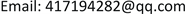1. 引言

u t + ( a + b u ) u u x + u x x x = 0 (1)

2. 预备知识2.1. 行波系统的奇点

d x d t = p ( x , y ) d y d t = q ( x , y ) (2)

J = ( p x p y q x q y )

① 如果 λ 1 和 λ 2 都是实数，且 λ 1 不等于 λ 2 ， λ 1 λ 2 > 0 ，那么 ( x 0 , y 0 ) 是一个结点，并且， λ 1 和 λ 2 都小于0时是稳定结点，都大于0时是非稳定结点；

② 如果 λ 1 和 λ 2 都是实数，且 λ 1 λ 2 < 0 ，那么 ( x 0 , y 0 ) 是一个鞍点；

③ 如果 λ 1 = λ 2 = λ 是实数，且 p y ( x 0 , y 0 ) 和 q x ( x 0 , y 0 ) 中至少有一个非零，那么 ( x 0 , y 0 ) 是一个退化结点，当 λ < 0 时是稳定的， λ > 0 时是不稳定的；

④ 如果 λ 1 = λ 2 = λ 是实数，且 p y ( x 0 , y 0 ) = q x ( x 0 , y 0 ) = 0 ，那么 ( x 0 , y 0 ) 是一个临界结点，当 λ < 0 时是稳定的， λ > 0 时是不稳定的；

⑤ 如果 λ 1 , 2 = α ± i β ， α ≠ 0 ，那么 ( x 0 , y 0 ) 是一个焦点，当 α < 0 时是稳定的， α > 0 时是不稳定的；

⑥ 如果 λ 1 , 2 = ± i β ，并且可以找到

d x d t = p ( x , y ) d y d t = q ( x , y )

u t = − c φ ′ (ξ)

u x = φ ′ (ξ)

u x x x = φ ‴ (ξ)

− c φ ′ ( ξ ) + a φ ( ξ ) φ ′ ( ξ ) + b φ 2 ( ξ ) φ ′ ( ξ ) + φ ‴ ( ξ ) = 0

− c φ ( ξ ) + a 2 φ 2 ( ξ ) + b 3 φ 3 ( ξ ) + φ ″ ( ξ ) = 0

d φ d ξ = y d y d ξ = c φ − a 2 φ 2 − b 3 φ 3 (3)

y = 0 , c φ − a 2 φ 2 − b 3 φ 3 = 0

2.2. 行波系统的首次积分

d φ d y = y c φ − a 2 φ 2 − b 3 φ 3

y ( ξ ) = ± 6 c φ 2 − 2 a φ 3 − b φ 4 + 12 C 6

H ( φ , y ) = 6 y 2 + 2 a φ 3 + b φ 4 − 6 c φ 2

3. 主要结果3.1. 参数不同取值下的奇点情况

c φ − a 2 φ 2 − b 3 φ 3 = 0

① a = 0 , b = 0 时，有 ( 0 , 0 ) 一个鞍点；

② a ≠ 0 , b = 0 时，有一个鞍点 ( 0 , 0 ) ，一个中心；

③ a = 0 , b > 0 时，有一个鞍点 ( 0 , 0 ) ，两个中心；

④ a ≠ 0 , b > 0 时，有一个鞍点 ( 0 , 0 ) ，两个中心；

⑤ − ( 3 a 2 ) / 16 c < b < 0 时，有一个中心，两个鞍点，其中一个鞍点是 ( 0 , 0 ) ；

⑥ b < − ( 3 a 2 ) / 16 c 时，只有一个鞍点 ( 0 , 0 ) ；

⑦ b = − ( 3 a 2 ) / 16 c 时，有一个鞍点 ( 0 , 0 ) ，和一个退化奇点。

c > 0 时的积分曲线图和数值模拟图见图1。

① a = 0 , b = 0 时，有 ( 0 , 0 ) 一个中心；

② a ≠ 0 , b = 0 时，有一个中心 ( 0 , 0 ) ，一个鞍点；

③ a = 0 , b < 0 时，有一个中心 ( 0 , 0 ) ，两个鞍点；

④ a ≠ 0 , b > 0 时，有一个中心 ( 0 , 0 ) ，两个鞍点；

⑤ 0 < b < − ( 3 a 2 ) / 16 c 时，有一个鞍点，两个中心，其中一个中心是 ( 0 , 0 ) ；

⑥ b > − ( 3 a 2 ) / 16 c 时，只有一个中心 ( 0 , 0 ) ；

⑦ b = − ( 3 a 2 ) / 16 c 时，有一个中心 ( 0 , 0 ) ，和一个退化奇点。

c < 0 时的积分曲线图和数值模拟图见图2。

① a = 0 , b = 0 时，有 ( 0 , 0 ) 一个退化奇点；

② b > 0 时，有 ( 0 , 0 ) 一个退化奇点，还有一个中心；

③ b < 0 时，有 ( 0 , 0 ) 一个退化奇点，还有一个鞍点。

c = 0 时的积分曲线图和数值模拟图见图3。

3.2. ab平面上的分支曲线和分支相图

H ( − 3 a − 9 a 2 + 48 b c 4 b , 0 ) = H ( 0 , 0 ) = 0

b = − a 2 6 c

4. 总结